# 9 4 factoring to solve quadratic equations What

• Slides: 8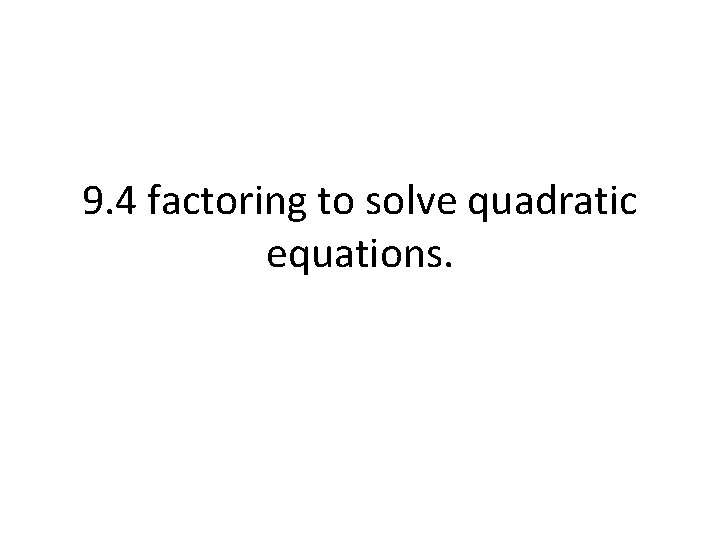9. 4 factoring to solve quadratic equations.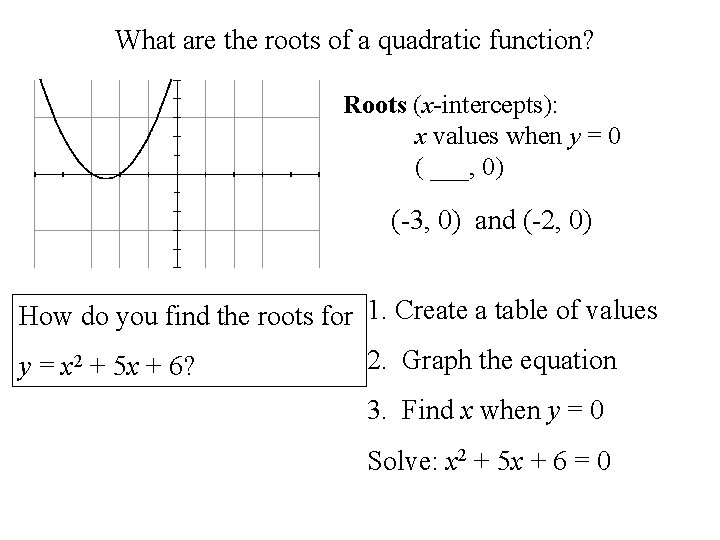What are the roots of a quadratic function? Roots (x-intercepts): x values when y = 0 ( ___, 0) (-3, 0) and (-2, 0) How do you find the roots for 1. Create a table of values y = x 2 + 5 x + 6? 2. Graph the equation 3. Find x when y = 0 Solve: x 2 + 5 x + 6 = 0Zero Product Rule List the factors of each. 6 36 x 2 + 5 x + 6 1 36 4 9 (x + 2) (x + 3) 2 3 2 18 6 6 3 12 0 If ab = 0, then a or b must be 0. Find the roots of: y = x 2 + 5 x + 6 = 0 (x + 2)(x + 3) = 0 x+2=0 x = -2 OR x+3=0 x = -3 Roots of x 2 + 5 x + 6 are (-2, 0) and (-3, 0).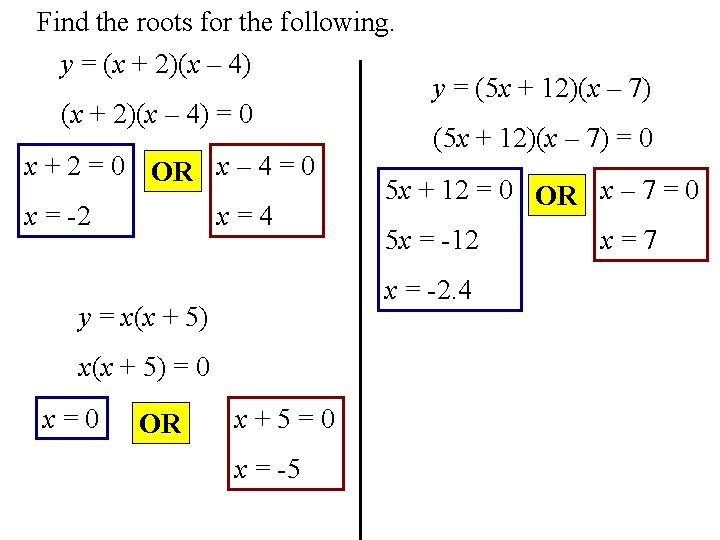Find the roots for the following. y = (x + 2)(x – 4) = 0 x + 2 = 0 OR x – 4 = 0 x = -2 x=4 x(x + 5) = 0 OR (5 x + 12)(x – 7) = 0 5 x + 12 = 0 OR x – 7 = 0 5 x = -12 x = -2. 4 y = x(x + 5) x=0 y = (5 x + 12)(x – 7) x+5=0 x = -5 x=7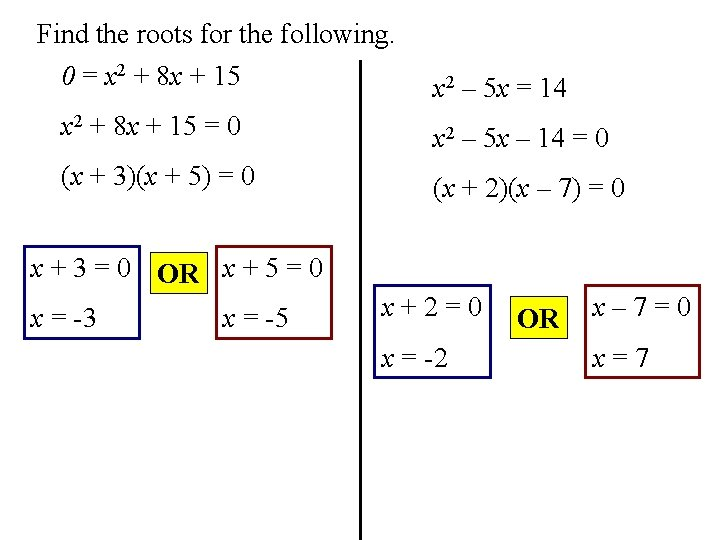Find the roots for the following. 0 = x 2 + 8 x + 15 x 2 – 5 x = 14 x 2 + 8 x + 15 = 0 x 2 – 5 x – 14 = 0 (x + 3)(x + 5) = 0 (x + 2)(x – 7) = 0 x + 3 = 0 OR x + 5 = 0 x = -3 x = -5 x+2=0 x = -2 OR x– 7=0 x=7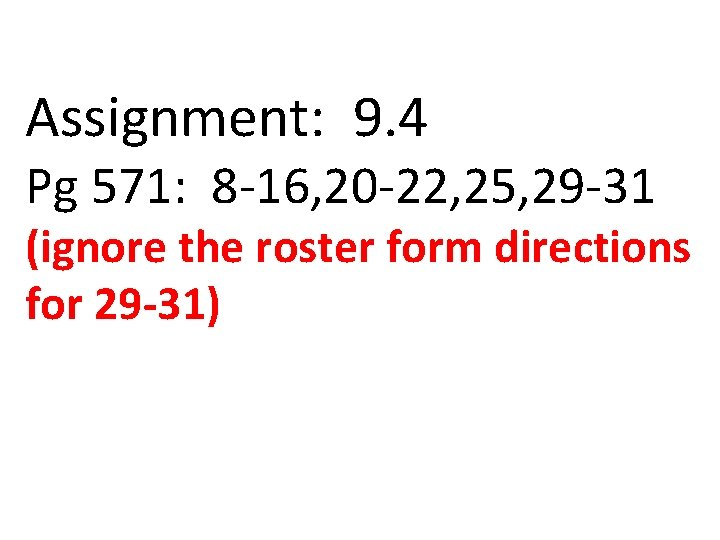Assignment: 9. 4 Pg 571: 8 -16, 20 -22, 25, 29 -31 (ignore the roster form directions for 29 -31)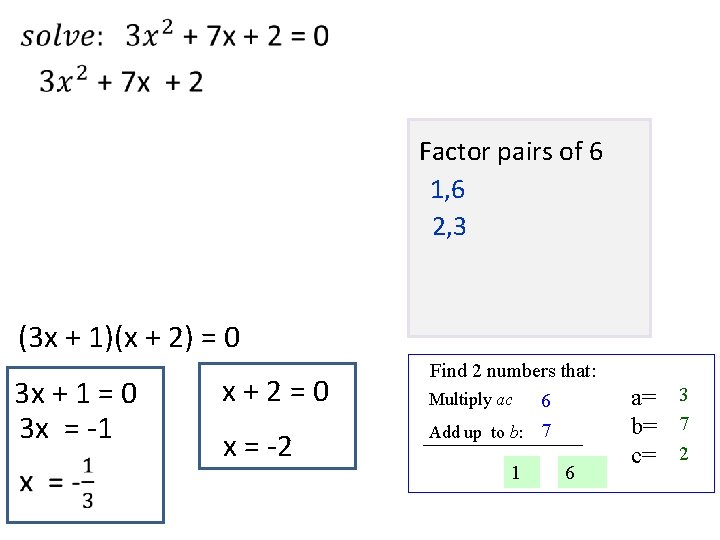Factor pairs of 6 1, 6 2, 3 (3 x + 1)(x + 2) = 0 3 x + 1 = 0 3 x = -1 x + 2 = 0 x = -2 Find 2 numbers that: Multiply ac 6 Add up to b: 7 1 6 a= 3 b= 7 c= 2Factor pairs of 6 1, 6 2, 3 (3 x + 1)(x + 2) = 0 3 x + 1 = 0 3 x = -1 x + 2 = 0 x = -2 Find 2 numbers that: Multiply ac 6 Add up to b: 7 1 6 a= 3 b= 7 c= 2